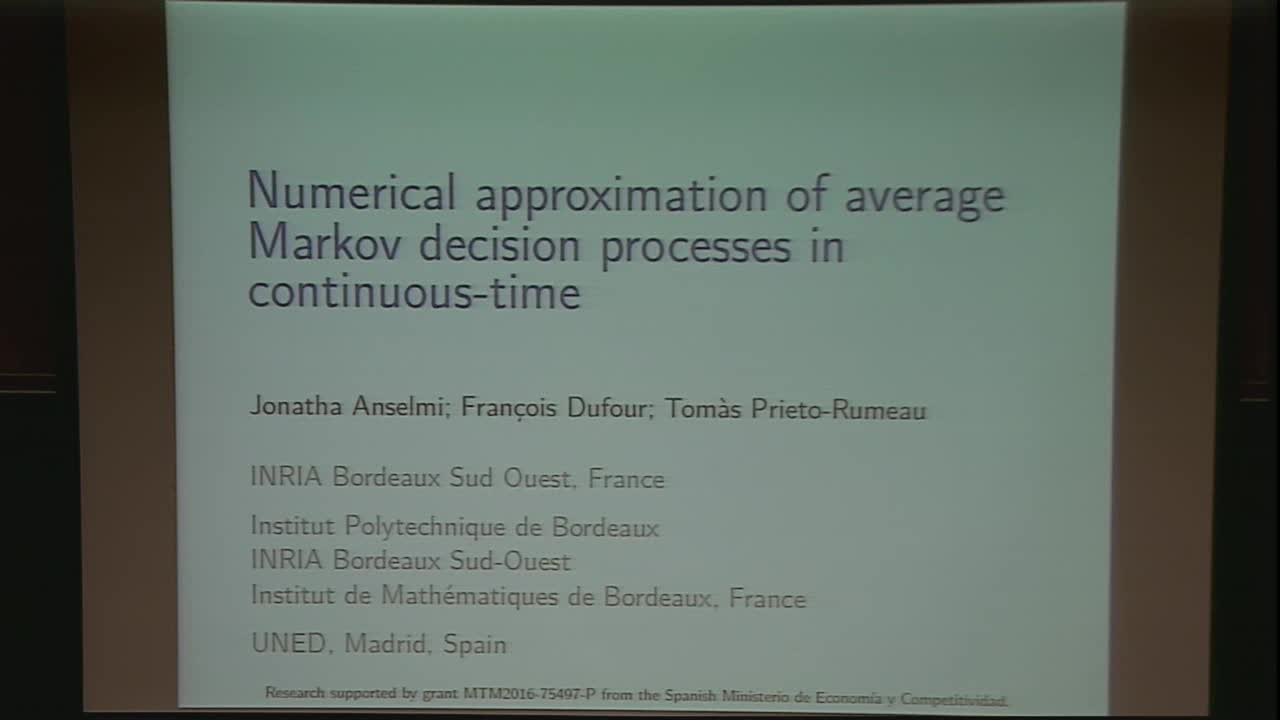## Numerical approximation of average Markov decision processes in continuous-time

May 8, 2018
IMA
Presenters: Francois Dufour

Watch Video

#### Abstract

We study a numerical approximation of the optimal long-run average cost of a continuous-time Markov decision process, with Borel state and action spaces, and with bounded transition and reward rates. Our approach uses a suitable discretization of the state and action spaces to approximate the original control model. The approximation error for the optimal average reward is then bounded by a combination of coefficients related to the discretization of the state and action spaces, namely, the Wasserstein distance between an underlying probability measure and a measure with finite support, and the Hausdorff distance between the original and the discretized actions sets. When approximating the underlying probability measure with its empirical probability measure we get convergence in probability at an exponential speed. An application to a queueing system is shown.